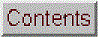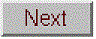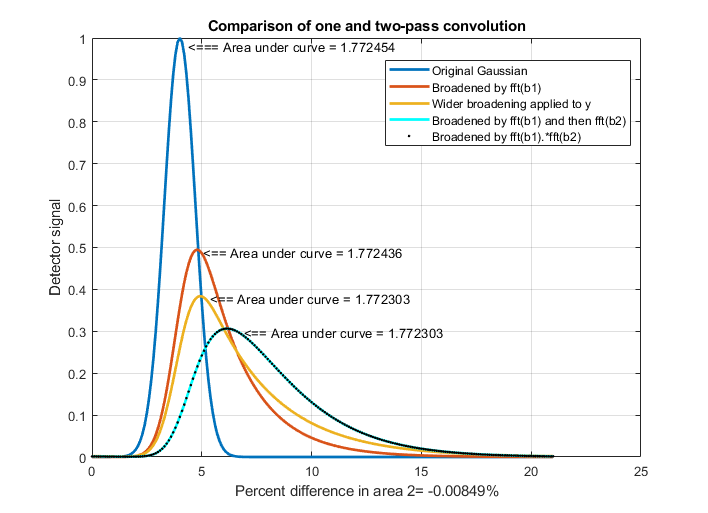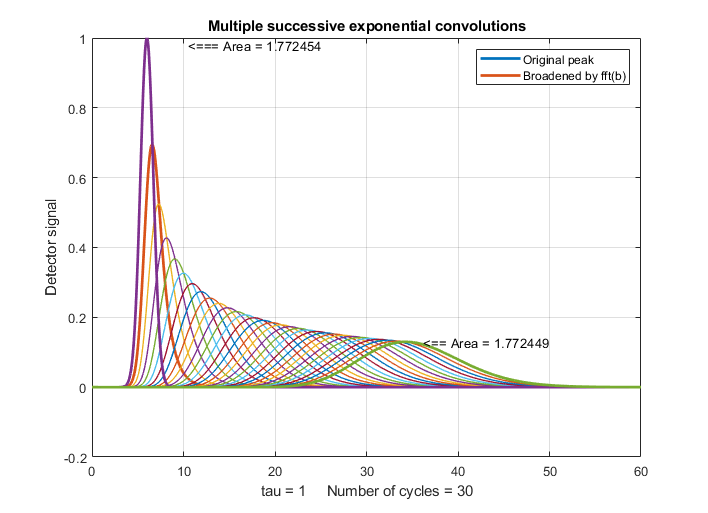[Introduction]  [Signal arithmetic]  [Signals and noise]   [Smoothing]   [Differentiation]  [Peak Sharpening]  [Harmonic analysis]   [Fourier convolution]  [Fourier deconvolution]  [Fourier filter]  [Wavelets]   [Peak area measurement]  [Linear Least Squares]  [Multicomponent Spectroscopy]  [Iterative Curve Fitting]  [Hyperlinear quantitative absorption spectrophotometry] [Appendix and Case Studies]  [Peak Finding and Measurement]  [iPeak]   [iSignal]  [Peak Fitters]   [iFilter]  [iPower]  [List of downloadable software]  [Interactive tools]Fourier Convolution

Convolution is an operation performed on two signals which involves multiplying one signal by a delayed or shifted version of another signal, integrating or averaging the product, and repeating the process for different delays. Convolution is a useful process because it accurately describes some effects that occur widely in scientific measurements, such as the influence of a frequency filter on an electrical signal or of the spectral bandpass of a spectrometer on the shape of a recorded optical spectrum, which cause the signal to be spread out in time and reduced in peak amplitude.

Fourier convolution is used here to determine how the optical spectrum in Window 1 (top left) will appear when scanned with a spectrometer whose slit function (spectral resolution) is described by the Gaussian function in Window 2 (top right). The Gaussian function has already been rotated so that its maximum falls at x=0, which is necessary to prevent the result from being shifted on the x-axis. The resulting convoluted optical spectrum (bottom center) shows that the two lines near x=110 and 120 will not be resolved but the line at x=40 will be partly resolved. Fourier convolution is used in this way to correct the analytical curve non-linearity caused by spectrometer resolution, in the "Tfit" method for hyperlinear absorption spectroscopy.

In practice, the calculation is usually performed by point-by-point multiplication of the two signals in the Fourier domain. First, the Fourier transform of each signal is obtained. Then the two Fourier transforms are multiplied point-by-point by the rules for complex multiplication and the result is then inverse Fourier transformed. Fourier transforms are usually expressed in terms of "complex numbers", with real and imaginary parts; if the Fourier transform of the first signal is a + ib, and the Fourier transform of the second signal is c + id, then the product of the two Fourier transforms is (a + ib)(c + id) = (ac - bd) + i(bc + ad). Although this seems to be  a round-about method, it turns out to be faster then the shift-and-multiply algorithm when the number of points in the signal is large. Convolution can be used as a powerful and general algorithm for smoothing and differentiation. Many computer languages will perform this operation automatically when the two quantities divided are complex.

Fourier convolution can also be used as a very general algorithm for the smoothing and differentiation of digital signals, by convoluting the signal with a (usually) small set of numbers representing the convolution vector. Smoothing is performed by convolution with sets of positive numbers, e.g. [1 1 1] for a 3-point boxcar. Convolution with [-1 1] computes a first derivative; [1 -2 1] computes a second derivative. Successive convolutions by Conv1 and then Conv2 is equivalent to one convolution with the convolution of Conv1 and Conv2.  First differentiation with smoothing is done by using a convolution vector in which the first half of the coefficients are negative and the second half are positive (e.g.[-1 -2 0 2 1]).

Simple whole-number convolution vectors:
Smoothing
3 point boxcar (sliding average) smooth
[1 1 1]
4 point boxcar (sliding average) smooth
[1 1 1 1]
3 point triangular smooth

[1 2 1]
5 point triangular smooth

[1 2 3 2 1]
5 point Gaussian smooth
[1 4 6 4 1]
7 point Gaussian smooth
[1 4 8 10 8 4 1]
9 point Gaussian smooth
[1 4 9 14 17 14 9 4 1]

Differentiation:
[-1 1]         First derivative
[1 -2 1]       Second derivative
[1 -3 3 -1]    Third derivative
[1 -4 6 -4 1]  Fourth derivative

Results of successive convolution by two
vectors Conv1 and Conv2:

Conv1               Conv2      Result
Triangular smooth
[1 1 1]     [1 1 1]  = [1 2 3 2 1]
Pseudo-Gaussian smooth
[1 2 1]     [1 2 1]  = [1 4 6 4 1]
2nd derivative
[-1 1]      [-1 1]   = [1 -2 1]
3rd derivative
[-1 1]      [1 -2 1] = [1 -3 3 -1]
Smoothed first derivative

[-1 1]      [1 1 1]  = [1 0 0 -1)
1st derivative gap-segment
[-1 1]      [1 2 1]  = [1 1 -1 -1)
Smoothed 1st derivative

same with more smoothing

[1 1 -1 -1] [1 2 1]  = [1 3 2 -2 -3 -1]

2nd derivative  gap-segment
[1 -2 1]    [1 2 1]  = [1 0 -2 0 1]

rectangle conv rectangle:
triangle or trapezoid
(depends on relative widths)
Gaussian conv  Gaussian:
Gaussian of greater width
Gaussian conv Lorentzian:
Something in between
Gaussian
Gaussian and Lorentzian

Spreadsheets can be used to perform "shift-and-multiply" convolution for small data sets (for example, MultipleConvolution.xls or MultipleConvolution.xlsfor Excel and MultipleConvolutionOO.ods for Calc), but for larger data sets the performance is much slower that Fourier convolution (which is much easier done in Matlab or Octave than in spreadsheets). Matlab and Octave have a built-in function for convolution of two vectors: conv. This function can be used to create very general type of filters and smoothing functions, such as sliding-average and triangular smooths. For example,

ysmoothed=conv(y,[1 1 1 1 1],'same')./5;

smooths the vector y with a 5-point unweighted sliding average (boxcar) smooth, and

ysmoothed=conv(y,[1 2 3 2 1],'same')./9;

smooths the vector y with a 5-point triangular smooth. The optional argument 'same' returns the central part of the convolution that is the same size as y. If that optional argument is "full", then the length of the result is ones less than the sum of the lengths of the two vectors.

Differentiation is carried out with smoothing by using a convolution vector in which the first half of the coefficients are negative and the second half are positive (e.g.[-1 0 1],[-2 -1 0 1 2], or [-3 -2 -1 0 1 2 3]) to compute a first derivative with increasing amounts of smoothing. The conv function in Matlab/Octave can easily be used to combine successive convolution operations, for example, a second differentiation followed by a 3-point triangular smooth:

>> conv([1 -2 1],[1 2 1])
ans =
1     0    -2     0     1

The next example creates an exponential trailing transfer function (c), which has an effect similar to a simple RC low-pass filter, and applies it to y.

c=exp(-(1:length(y))./30);
yc=conv(y,c,'full')./sum(c);

In each of the above three examples, the result of the convolution is divided by the sum of the convolution transfer function, in order to insure that the convolution has a net gain of 1.000 and thus does not effect the area under the curve of the signal. This makes the mathematical operation closer to the physical convolutions that spread out the signal in time and reduce the peak amplitude, but conserve the total energy in the signal, which for a peak-type signal is proportional to the area under the curve.

Alternatively, you could perform the convolution yourself without using the built-in Matlab/Octave "conv" function by multiplying the Fourier transforms of y and c using the "fft.m" function, and then inverse transform the result with the "ifft.m" function. The results are essentially the same as the Matlab "conv" function with the 'same' option. and the elapsed time is actually slightly faster than using the conv function. However, c must be centered at zero and  zero-filled to match the size of yc because the point-by-point multiplication or division of two vectors requires that they have the same length.  The "conv" function performs any required zero filling automatically.

yc=ifft(fft(y).*fft(c));

When using convolution for the purposes of smoothing, it's usually desired that the area under the curve y remain the same after smoothing, This is easily ensured by dividing by the sum of the members of c:

yc=ifft(fft(y).*fft(c))./sum(c);

GaussConvDemo.m shows that a Gaussian of unit height convoluted with a  Gaussian of the same width is a Gaussian with a height of 1/sqrt(2) and  a width of sqrt(2) and of equal area to the original Gaussian. (Figure 2 shows an attempt to recover the original y from the convoluted yc by using the deconvgauss function). You can optionally add noise in line 9 to show how convolution smooths the noise and how deconvolution  restores it. Requires gaussian.m, peakfit.m and deconvgauss.m in path.

iSignal version 5.7 has a Shift-V keypress that displays the menu of Fourier convolution and deconvolution operations that allow you to convolute a Gaussian or exponential function with the signal, or to deconvolute a Gaussian or exponential function from the signal, and asks you for the width or the time constant (in X units).

Multiple sequential convolution. In the real world, signal broadening mechanisms are not always reducible to a single convolution. Sometimes two or more convolution mechanisms may be in play at the same time.A good example of this occurs in the technique of twin-column recycling separation process (TCRSP), a novel chromatography technique in which the injected sample is recycled back to the two columns for obtaining better and better resolution, allowing chromatographers to solve challenging separation problems caused by the partition coefficients for the components being too similar and/or too low column efficiencies [reference 90]. In TCRSP, after the sample is separated by the first column, it flows into the second identical column, and after that separation, switching valves connect it back into the first column. That cycle repeats as many times as required. Each pass through a column increases the separation between the components slightly, so that with a sufficiently large number of cycles, very similar substances can be separated. As described in a later section, chromatographic separations often involve broadening of the peaks by asymmetrical mechanisms, usually modeled as an exponentially modified Gaussian (EMG). Any broadening that occurs in the first pass will occur repeatedly in the subsequent passes. The net result will be a final peak shape that cannot be described by a single convolution. The success of the TCRSP technique depends on the fact that the separation between the components increases faster than the width increase caused by the successive convolutions of broadening mechanisms. But multiple sequential convolutions produce results that differ from a single large convolution.This is demonstrated by the simple example of two sequential exponential convolutions applied to a Gaussian, as shown in the figure on the left, generated by a Matlab/Octave script. The blue curve is the original Gaussian. The red curve is the result of a single convolution by an exponential function whose time constant tau is 2. The cyan curve is the result of two successive convolutions with that same tau. The orange curve is an attempt to duplicate that with a single wider convolution of tau equal to 3. That attempt fails; the result is a poor match to the cyan curve. In fact, experiments show that no single wider exponential convolution can match the result of two (or more) successive convolutions; the shape is fundamentally different. Multiple exponential convolutions result in a less asymmetrical peak, more shifted to larger x values. On the other hand, a single convolution by a function that is the product of the Fourier transforms of the two separate functions does work (black dots). With greater numbers of successive convolutions, the peaks become progressively  more symmetrical and more Gaussian as the number of cycles increases, as demonstrated for 30 cycles by the figure on the right, which was created by this Matlab/Octave script (where you can choose the number of convolutions in line 20).March 2021. This page is part of "A Pragmatic Introduction to Signal Processing", created and maintained by Prof. Tom O'Haver , Department of Chemistry and Biochemistry, The University of Maryland at College Park. Comments, suggestions and questions should be directed to Prof. O'Haver at toh@umd.edu.
Unique visits since May 17, 2008: